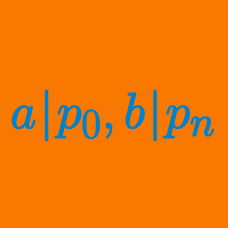Algebra

# Rational Root Theorem

What is the sum of all the rational roots of $x^{7}-2x^{6}+7x-14?$

If a polynomial of degree $11$ has integer coefficients, a leading coefficient of $3$, and constant term $11$, what is the maximum number of distinct rational roots of this polynomial?

What is the sum of the elements of the set $X=\{3, 11, 26\}$ that can be roots of the following polynomial for some integers $m$ and $n$: $x^{6}+mx^{4}+nx-33?$

What is the product of all the distinct rational roots of the polynomial $2x^3-9x^2+12x-4?$

What is the sum of all real roots of the polynomial $x^3+2x^2+2x-51?$

×